# Isotopy of immersions

## Definition

Suppose$M, N$ are smooth manifolds of dimensions$m, n$ and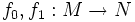$f_0,f_1: M \to N$ are two smooth immersions. An isotopy from$f_0$ to$f_1$ is a map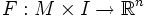$F:M \times I \to \R^n$ such that the following hold:

•$F$ is a smooth map
•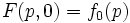$F(p,0) = f_0(p)$ and$F(p,1) = f_1(p)$: In other words$F$ is a homotopy from$f_0$ to$f_1$
• For each$t$, the map from$M$ to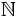$\N$ given by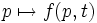$p \mapsto f(p,t)$ is an immersion